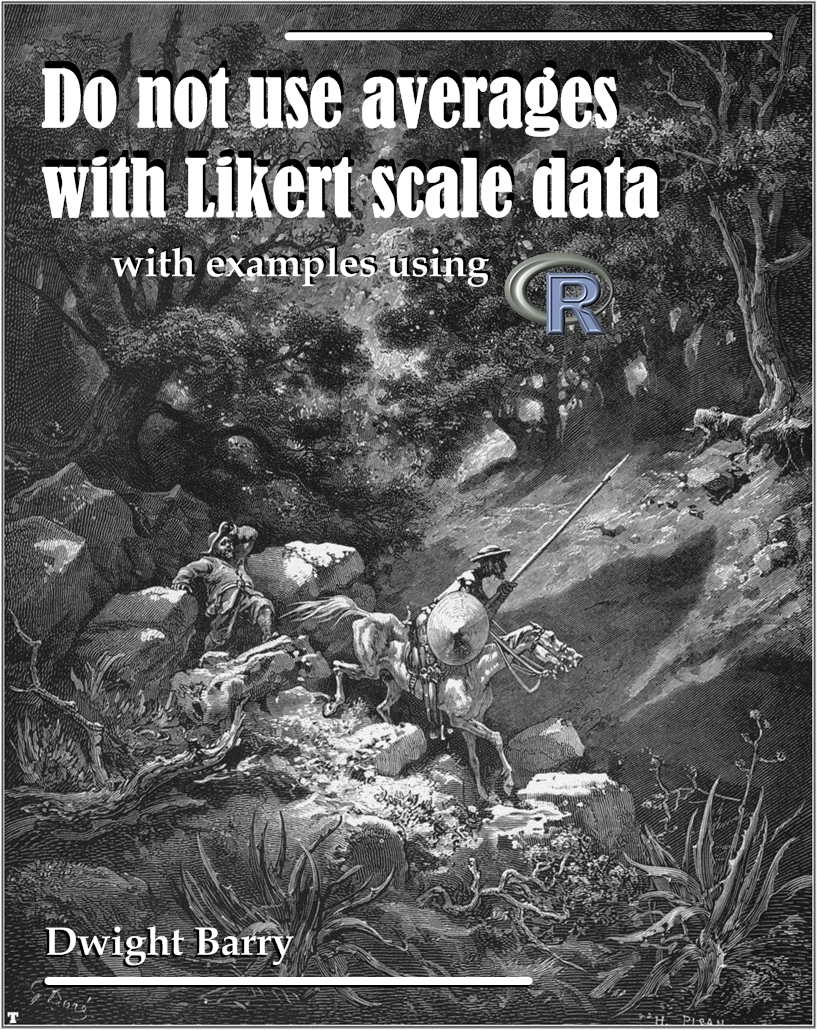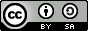This is a short overview of why averages don’t work well for evaluating Likert scale or other ordinal-scale data, and what to do instead, with examples using R. While the examples are focused on healthcare surveys, the lessons apply to any use of ordinal scale data.

Note: all of the data in this document is fake, created specifically to illustrate particular points.

Cover image: Gustave Doré, 1863. Illustration 12 for Cervantes’s Don Quixote. Public Domain.R packgaes

#### Packages ####
library(grid)
library(nnet)
library(coin)
library(boot)
library(simpleboot)
library(knitr)
library(ggplot2)
library(dplyr)
library(AICcmodavg)
library(polycor)
library(likert)
library(MASS)
library(ordinal)

Data

#### Basic example data set ####
person = c('A','B','C','D','E','F')

# Original
year1 = c(5,4,4,4,4,4)
year2 = c(2,5,5,5,5,4)
year3 = c(3,5,5,5,5,3)
year4 = c(1,5,5,5,5,5)

# A more obvious version
# year1 = c(3,3,3,3,3,3)
# year2 = c(4,4,4,2,2,2)
# year3 = c(5,4,3,3,2,1)
# year4 = c(5,5,5,1,1,1)

ex_1 = data.frame(person, year1, year2, year3, year4)

ex_1_long = reshape2::melt(ex_1)

#### Larger example data set ####

set.seed(29)

md = data.frame(Group = as.character("MD"),
Response1 = ordered(sample(1:5, 100, replace=T, prob=c(.1,.1,.1,.2,.5))),
Response2 = ordered(sample(1:5, 100, replace=T, prob=c(.1,.3,.3,.25,.15))))
rn = data.frame(Group = as.character("RN"),
Response1 = ordered(sample(1:5, 100, replace=T, prob=c(.1,.1,.5,.2,.1))),
Response2 = ordered(sample(1:5, 100, replace=T, prob=c(.1,.15,.45,.15,.15))))

both = rbind(md, rn)

make_NAs = sample(1:200, 15, replace=F)
both$Response1[make_NAs] = NA make_NAs2 = sample(1:200, 15, replace=F) both$Response2[make_NAs2] = NA

# Add question names to data
names(both) = c("EmployeeType",
"My team works well together.",
"I have the tools I need to do my job.")

#### Dashboarding pain scores example ####

# Create list for random pain scores
pain_list = list()

for(i in 1:24){
set.seed(i)
pain_level = ordered(sample(c("Low", "Medium", "High"), size = sample(10:30),
replace = T, prob = c(.15, .45, .40)), levels = c("Low", "Medium", "High"))
pain_list[[i]] = table(pain_level)
}

# Unlist into a data frame
pain_df = data.frame(matrix(unlist(pain_list), nrow=24, byrow=T))
colnames(pain_df) = c("Low", "Medium", "High")

pain_scores = data.frame(Month = seq(as.Date("2014-10-01"), by = "month",
length.out = 24), pain_df)

# Melt into long form, I really should learn tidyr
pain_scores = reshape2::melt(pain_scores, id.vars = "Month",
variable.name = "Pain_Group", value.name = "Count")

# Summarize to get counts and percentages
surgeries_pain = pain_scores %>%
group_by(Month) %>%
mutate(Surgeries = sum(Count), percent = (Count / sum(Count)),
cumsum = cumsum(percent))

#### For use with chi-square and regression models ####

# Get rid of NAs
both2 = na.omit(both)

# Rename columns to something more R-friendly
names(both2) = c("EmployeeType", "Teamwork", "Tools")

# Reverse the levels so 5 will be at top of mosaic plot
both2$Teamwork = ordered(both2$Teamwork, levels = c("5", "4", "3", "2", "1"))

# Make a table object
both2_tab = xtabs(~ both2$EmployeeType + both2$Teamwork)

# For multinomial and prop odds models
both3 = both2

# Bring axis back to normal
both3$Teamwork = ordered(both3$Teamwork, levels = c("1", "2", "3", "4", "5"))

# Data frame for proportional odds regression
Teamwork_tab_long = both3[,1:2] %>%
group_by(EmployeeType, Teamwork) %>%
summarize(Count = n())

# Function to turn counts into rows I found laying around the web somewhere
countsToCases = function(x, countcol = "Count") {
# Get the row indices to pull from x
idx = rep.int(seq_len(nrow(x)), x[[countcol]])
# Drop count column
x[[countcol]] = NULL
# Get the rows from x
x[idx, ]
}

# Make a data frame for prop odds
Teamwork_tab_long$Teamwork_Group = as.numeric(Teamwork_tab_long$Teamwork)
Teamwork_tab_long$Teamwork = ordered(Teamwork_tab_long$Teamwork)
tab_df = data.frame(countsToCases(Teamwork_tab_long, countcol="Count"))